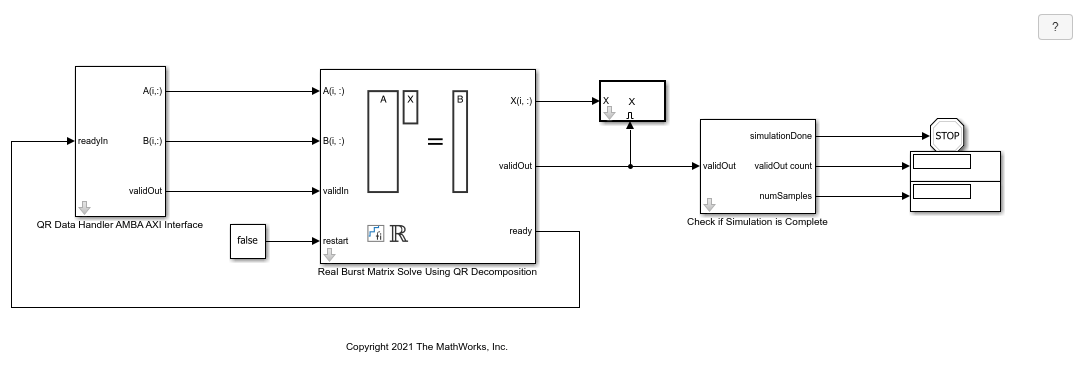Main Content

# Implement Hardware-Efficient Real Burst Matrix Solve Using QR Decomposition

This example shows how to implement a hardware-efficient least-squares solution to the real-valued matrix equation AX=B using the Real Burst Matrix Solve Using QR Decomposition block.

### Define Matrix Dimensions

Specify the number of rows in matrices A and B, the number of columns in matrix A, and the number of columns in matrix B.

```m = 50; % Number of rows in matrices A and B n = 10; % Number of columns in matrix A p = 1; % Number of columns in matrix B ```

### Generate Random Least-Squares Matrices

For this example, use the helper function `realRandomLeastSquaresMatrices` to generate random matrices A and B for the least-squares problem AX=B. The matrices are generated such that the elements of A and B are between -1 and +1, and A has rank r.

```rng('default') r = 3; % Rank of A [A,B] = fixed.example.realRandomLeastSquaresMatrices(m,n,p,r); ```

### Select Fixed-Point Data Types

Use the helper function `realQRMatrixSolveFixedpointTypes` to select fixed-point data types for input matrices A and B, and output X such that there is a low probability of overflow during the computation. For more information about how datatypes are selected, see the document FixedPointMatrixLibraryDatatypesExample.pdf in the current directory.

```max_abs_A = 1; % max(abs(A(:)) max_abs_B = 1; % max(abs(B(:)) f = 24; % Fraction length (bits of precision) T = fixed.example.realQRMatrixSolveFixedpointTypes(m,n,max_abs_A,max_abs_B,f); A = cast(A,'like',T.A); B = cast(B,'like',T.B); OutputType = fixed.extractNumericType(T.X); ```

### Open the Model

```model = 'RealBurstQRMatrixSolveModel'; open_system(model); ```The Data Handler subsystem in this model takes real matrices A and B as inputs. The `ready` port triggers the Data Handler. After sending a true `validIn` signal, there may be some delay before `ready` is set to false. When the Data Handler detects the leading edge of the `ready` signal, the block sets `validIn` to true and sends the next row of A and B. This protocol allows data to be sent whenever a leading edge of the `ready` signal is detected, ensuring that all data is processed.

### Set Variables in the Model Workspace

Use the helper function `setModelWorkspace` to add the variables defined above to the model workspace. These variables correspond to the block parameters for the Real Burst Matrix Solve Using QR Decomposition block.

```numSamples = 1; % Number of sample matrices fixed.example.setModelWorkspace(model,'A',A,'B',B,'m',m,'n',n,'p',p,... 'numSamples',numSamples,'OutputType',OutputType); ```

### Simulate the Model

```out = sim(model); ```

### Construct the Solution from the Output Data

The Real Burst Matrix Solve Using QR Decomposition block outputs data one row at a time. When a result row is output, the block sets `validOut` to true. The rows of X are output in the order they are computed, last row first, so you must reconstruct the data to interpret the results. To reconstruct the matrix X from the output data, use the helper function `matrixSolveModelOutputToArray`.

```X = fixed.example.matrixSolveModelOutputToArray(out.X,n,p,numSamples); ```

### Verify the Accuracy of the Output

To evaluate the accuracy of the Real Burst Matrix Solve Using QR Decomposition block, compute the relative error.

```relative_error = norm(double(A*X - B))/norm(double(B)) %#ok<NOPTS> ```
```relative_error = 3.5192e-06 ```

## SupportGet trial now